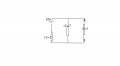# using power transfer theorem

#### uksparky

Joined Jan 6, 2013
3
using thevenin's theorem on this circuit
calculate PD across terminal AB and ??
the impedance looking in from AB ???

then using maximum power transfer ;
1)Calculate the P.D across AB ?
2)the current with rl connected ?
3)the maximum power transferred in the circuit ?
please can someone help me calculate this in the simplest format as i don't no where to start to get these results

r1=3 ohms
r2=7ohms
rl=? this is across terminal AB
V=20v#### WBahn

Joined Mar 31, 2012
26,451
This is the Homework Help, not the Homework Do It For Me, forum.

You need to show some effort to solve the problem. If nothing else, discuss what you know about what you are trying to find and the concepts that apply to the problem, even if you aren't sure how to actually apply them.

#### uksparky

Joined Jan 6, 2013
3
ok sorry i did this but not sure if right Using Thevenin’s Theorem calculate PD across terminal AB:
Short all voltage sources
3x7/(3+7) =21/10=2.1Ω
I=V/R add the voltage back in now 20/10=2A
as this is a series circuit the current remains the same
so putting the voltage back in we have 20v/2A=10ohms and 10-2.1 ohms=7.9ohms RL
i cant do any more without help im not after the answer just the method ie v*i+z ETC

#### WBahn

Joined Mar 31, 2012
26,451
I agree that the Thevenin resistance is 2.1Ω. You need to get in the habit (whether the teacher or the text does so or not) of tracking and using your units throughout your work, so this computation should be presented something like:

Rth = R1||R2
= (R1*R2)/(R1+R2)
= (3Ω*7Ω)/(3Ω+7Ω)
= (21/10)Ω^2/Ω
= 2.1Ω

Now, it would have been reasonable to omit a few of the minor steps (i.e., do them mentally), but keep in mind that a grader is not a mind reader and must assign partial credit based on the work presented. The more you give them, and the more organized and easy to follow it is, the more partial credit you will receive. Trust me, it pays off. Not only do you get significantly more partial credit, but you will catch the majority of your own errors and get full credit more of the time as well.

But you next line shows major misconceptions about fundamental circuits. Upon what basis do you claim I = 20V/10Ω? Do not say Ohm's Law. Ohm's Law is a relation between the resistance of a resistor to the voltage across THAT resistor and the current through THAT resistor. The 20V does NOT appear as the voltage across the 10Ω resistor. When the load resistor, Rload, is in place, it is the voltage across the series combination of Rth and Rload.

This is why it is important to present your work here, because the only way I could even guess that this was a problem for you was seeing enough of your work for this problem to become apparent.

#### uksparky

Joined Jan 6, 2013
3
Using Thevenin’s Theorem calculate PD across terminal AB:
Short all voltage sources and find open cuircuit voltage
r2/(r1+r2)=Vth 7/(3+7)=0.7Vth
Find thevenin resistance
3x7/(3+7) =21/10=2.1Ωth
Using ohms law
0.7/2.1=0.3333333Ath
Il=(Vth)/Rl and as they are in series the resistances are the same so RL=2.1 ohms
2.1 ohms as above section 3
and the potential difference is
????
here is what i have done now is this ok and how do i find PD

#### WBahn

Joined Mar 31, 2012
26,451
Using Thevenins Theorem calculate PD across terminal AB:
Short all voltage sources and find open cuircuit voltage
If all the voltage sources are shorted, then how is there any open circuit voltage at all?

r2/(r1+r2)=Vth 7/(3+7)=0.7Vth
The left hand side has no units at all while the right hand side has units of voltage. You KNOW it's wrong!

Find thevenin resistance
3x7/(3+7) =21/10=2.1Ωth
If you can't be bothered to track your units, then I can't be bothered to continue trying to help you.

Using ohms law
0.7/2.1=0.3333333Ath
On what basis do you claim that 0.7 (what are the units!!!!) appears across the equivalent resistance? You are still just grabbing any handy voltage and any hand resistance to get something that has the appearance of being a current. It's like me saying that my car has a 12V battery and the toaster in my kitchen has a heating element resistance of 2Ω and concluding that my radio is drawing 6A.